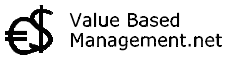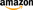## AutoRegressive Integrated Moving Average# ARIMA

## Summary of AutoRegressive Integrated Moving Average. Abstract

 Although nobody can really look into the future, modern statistical methods, econometric models and business intelligence software go a long way in helping businesses forecast and estimate what is going to happen in the future.   ARIMA stands for AutoRegressive Integrated Moving Average. The ARIMA Time Series Analysis uses lags and shifts in the historical data to uncover patterns (e.g. moving averages, seasonality) and predict the future. The ARIMA model was first developed in the late 60s but was systemized by Box and Jenkins in 1976. A can be more complex to use than other statistical forecasting techniques, although when implemented properly can be quite powerful and flexible.   A is a method for determining two things: 1. How much of the past should be used to predict the next observation (length of weights) and 2. The values of the weights. For example y(t)= 1/3 * y(t-3) + 1/3 * y(t-2) + 1/3 * y(t-1) is an ARIMA model; another one is y(t)= 1/6 * y(t-3) + 4/6 * y(t-2) + 1/6 * y(t-1) Thus the correct A model requires identification of the right number of lags and the coefficients that should be used. A model identification uses autoregressions to IDENTIFY the underling model. Care must be taken to robustly IDENTIFY and ESTIMATE PARAMETERS as outliers (pulses, level shifts , local time trends ) can wreak havoc.   Book: Alan Pankratz - Forecasting with Univariate Box Jenkins Models : Concepts and Cases -Book: Jeffrey Wooldridge - Introductory Econometrics: A Modern Approach -Compare with ARIMA: Regression Analysis  |  Dynamic Regression  |  Exponential Smoothing